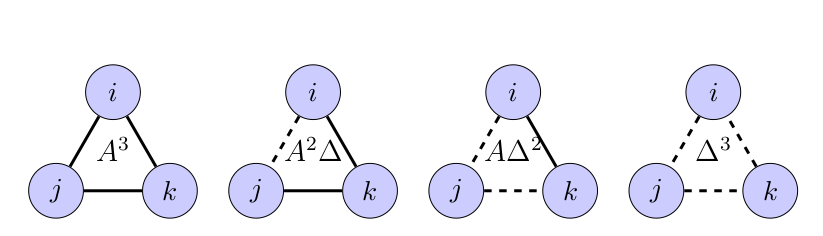A streaming triangle counting algorithm can be derived from the static definition

# Deriving Streaming Graph Algorithms from Static Definitions

### Abstract

Increasing volumes of data and the desire for real-time query capability make the development of efficient streaming algorithms for data analytics valuable. Streaming graph algorithms that avoid unnecessary recomputation through clever application of data dependency analysis are often more complex to derive than their static counterparts. This paper discusses a method to derive algorithms for streaming graph analysis from static formulations Combining tuned graph algorithms building blocks with an appropriate functional language, a graph query planner should be able to correctly implement most static and streaming versions of an algorithm from a single mathematical formulation. We provide a detailed analysis for the case of updating triangle counts in a streaming graph using linear algebra and an experimental evaluation in Julia.

Publication
International Parallel & Distributed Processing Symposium
Date
Links